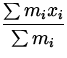# Center of Gravity

Definition: Center of Gravity

The center of gravity of a collection of masses is the point where all the weight of the object can be considered to be concentrated. If (xcg,ycg) are the coordinates of the centre of gravity of a collection of point masses m1 , m2 , etc, located at coordinates ( x1,y1 ), ( x2,y2 ), respectively, then:

 (m1 + m2 + ..)gxcg = m1gx1 + m2gx2 +... (5) (m1 + m2 + ..)gycg = m1gy1 + m2gy2 +... (6)
Equations (8.6) and (8.6) imply that the torque about the origin would be the same if the entire weight acted through the center of gravity instead of acting through the individual masses.

Solving for the x -coordinate of the center of gravity:

 xcg =. (7)

Similary, the y-coordinate of the centre of gravity is:

 ycg =. (8)

Note:

• Eqs(8.6,8.6) also imply that the torque due to gravity about the center of gravity is zero. The force of gravity, F = mg , acts through the center of gravity so there is no moment arm and therefore no torque due to gravity about the center of gravity.
• An extended body can be treated in exactly the same way simply by considering it to be made up of a collection of smaller masses stuck together".
• The centre of gravity is not necessarily inside the object.
• In problems involving extended bodies and gravity, one can impose the equilibrium condition by assuming that the entire weight of the bodies acts through the centre of gravity.
• In order to balance an object against gravity with a single force, that force must lie in a vertical line that runs through the centre of gravity.
• For objects with symmetry, the center of gravity is always located along the axis of symmetry.
• The center of gravity of an extended body or system of masses is also distinguished by the fact that it will remain at rest or moving at constant velocity unless the body is acted on by a net external force.Next: Relation Between Torque and Up: Rotational Equilibrium and Dynamics Previous: Another Condition for Equilibrium